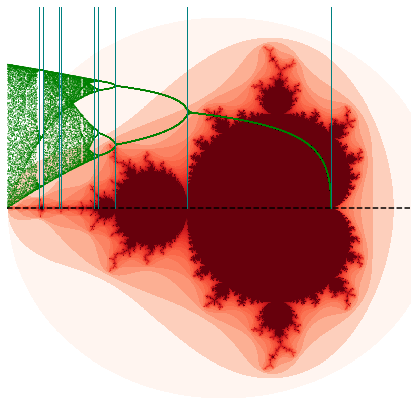# Verhulst mandelbrot¶

## verhulst mandelbrot¶

Most examples work across multiple plotting backends, this example is also available for:

Example showing how bifurcation diagram for the logistic map relates to the Mandelbrot set according to a linear transformation. Inspired by this illustration on Wikipedia.

In :
from itertools import islice

import numpy as np
import holoviews as hv
from holoviews import opts

hv.extension('matplotlib')## Defining Mandelbrot and Logistic Map¶

In :
# Area of the complex plane
bounds = (-2,-1.4,0.8,1.4)
# Growth rates used in the logistic map
growth_rates = np.linspace(0.9, 4, 1000)
# Bifurcation points
bifurcations = [1, 3, 3.4494, 3.5440, 3.5644, 3.7381, 3.7510, 3.8284, 3.8481]

def mandelbrot_generator(h,w, maxit, bounds=bounds):
"Generator that yields the mandlebrot set."
(l,b,r,t) = bounds
y,x = np.ogrid[b:t : h*1j, l:r:w*1j]
c = x+y*1j
z = c
divtime = maxit + np.zeros(z.shape, dtype=int)
for i in range(maxit):
z  = z**2 + c
diverge = z*np.conj(z) > 2**2
div_now = diverge & (divtime==maxit)
divtime[div_now] = i
z[diverge] = 2
yield divtime

def mandelbrot(h,w, n, maxit):
"Returns the mandelbrot set computed to maxit"
iterable =  mandelbrot_generator(h,w, maxit)
return next(islice(iterable, n, None))

def mapping(r):
"Linear mapping applied to the logistic bifurcation diagram"
return (r /2.0) * ( 1 - (r/2.0))

def logistic_map(gens=20, init=0.5, growth=0.5):
population = [init]
for gen in range(gens-1):
current = population[gen]
population.append(current * growth * (1 - current))
return population


# Plot¶

In :
bifurcation_diagram = hv.Points([(mapping(rate), pop) for rate in growth_rates for
(gen, pop) in enumerate(logistic_map(gens=200, growth=rate))
if gen>=100])  # Discard the first 100 generations to view attractors more easily

img = hv.Image(mandelbrot(800,800, 45, 46).copy(), bounds=(-2, -1.4, 0.8, 1.4))

vlines = hv.Overlay([hv.Curve([(mapping(pos),0), ((mapping(pos),1.4))]) for pos in bifurcations])

(img * bifurcation_diagram * hv.HLine(0) * vlines).opts(
opts.Curve(color='teal', linewidth=1),
opts.HLine(color='k', linestyle='--'),
opts.Image(cmap='Reds', fig_size=200, logz=True, xaxis=None, yaxis=None),
opts.Points(s=1, color='g'))

Out: